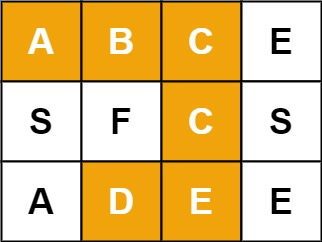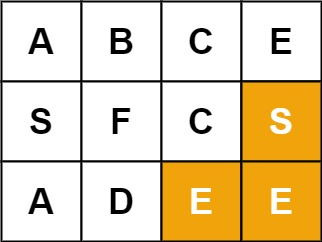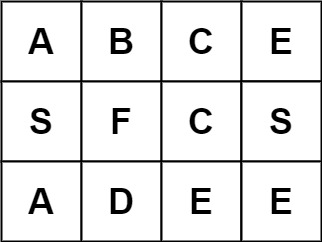# 79. Word Search | LeetCode Solution

## 79. Word Search | LeetCode Solution

Given an `m x n` grid of characters `board` and a string `word`, return `true` if `word` exists in the grid.

The word can be constructed from letters of sequentially adjacent cells, where adjacent cells are horizontally or vertically neighboring. The same letter cell may not be used more than once.

Example 1:```Input: board = [["A","B","C","E"],["S","F","C","S"],["A","D","E","E"]], word = "ABCCED"
Output: true
```

Example 2:```Input: board = [["A","B","C","E"],["S","F","C","S"],["A","D","E","E"]], word = "SEE"
Output: true
```

Example 3:```Input: board = [["A","B","C","E"],["S","F","C","S"],["A","D","E","E"]], word = "ABCB"
Output: false
```

Constraints:

• `m == board.length`
• `n = board[i].length`
• `1 <= m, n <= 6`
• `1 <= word.length <= 15`
• `board` and `word` consists of only lowercase and uppercase English letters.
class Solution {
public:
bool solve(vector<vector<char>>& v, string &w,int i,int j)
{
if(w.size()==0)
{
return 1;
}
if(i<0 || j<0 || i>=v.size() || j>=v.size() || v[i][j]=='0' || v[i][j]!=w)
{
return false;
}

char x=v[i][j];
v[i][j]='0';
w=w.substr(1);

bool a= solve(v,w,i+1,j) || solve(v,w,i-1,j) || solve(v,w,i,j-1) || solve(v,w,i,j+1);
v[i][j]=x;
w=x+w;
return a;
}
bool exist(vector<vector<char>>& v, string w) {
vector<vector<bool>> vis(v.size(),vector<bool>(v.size(),0));
for(int i=0;i<v.size();i++)
{
for(int j=0;j<v[i].size();j++)
{
if(solve(v,w,i,j))
return 1;
}
}
return 0;
}
};